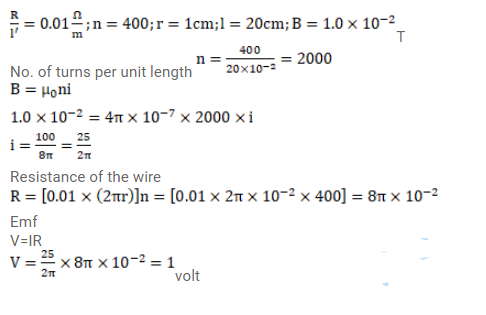# A copper wire having resistance 0.01ohm

Question:

A copper wire having resistance $0.01$ ohm in each meter is used to wind a 400 -turn solenoid of radius $1.0 \mathrm{~cm}$ and length $20 \mathrm{~cm}$. Find the emf of a battery which when connected across the solenoid will cause a magnetic field of $1.0 \times 10^{-2}$ T near the center of the solenoid.

Solution: